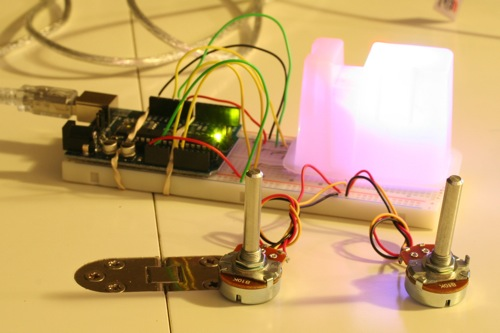# Theory and Practice of Tangible User Interfaces

### Announcements

November 24, 2007
Reading for November 27th, are now posted. Enjoy!

October 2, 2007
To upload your thoughtless acts, create a new assignment page like any other lab. You'll see "Thoughtless Acts" listed as one of the assignment options.

May 24, 2008
This site has been archived and is no longer editable. Stay tuned for the next version, coming in the fall!

Project Members:
Ken-ichi Ueda

### Description

Two pots control three LEDs. LEDs blink in a syncopated rhythm, and the
pots control brightness and rhythm speed. One drawback is that pot
input only takes affect after the beat has completed.

### Components Used

• LEDs
• Potentiometers
• Resistors

### Arduino Code

`/*`
`  LightBeat`
`  `
`  Two pots control three LEDs.  LEDs blink in a syncopated rhythm, and the `
`  pots control brightness and rhythm speed.  One drawback is that pot`
`  input only takes affect after the beat has completed.`
`  `
`  By Ken-ichi Ueda`
`*/`
`int pinR = 9;`
`int pinG = 10;`
`int pinB = 11;`
`int pins[] = {pinR, pinG, pinB};`
`int potA = 0;`
`int potB = 1;`
`int valA = 0; // brightness`
`int valB = 0; // rhythm speed`
`int i = 0; // loop counter`
`int minWait = 100;`
`int maxWait = 1000;`
`int fadeT = 5;`
`//int actPinIndex = 0; // active pin in the rhythm`
`int actPin = pinR;`
`int const meter = 9;`
`float rhythm = {1,    1,    2,    1.5,  0.5,  0.5,  1,    0.25, 0.25};`
`int sequence = {pinR, pinR, pinB, pinR, pinR, pinB, pinR, pinG, pinG};`
`int DEBUG = 1;`
`void setup() {`
`  pinMode(pinR, OUTPUT);`
`  pinMode(pinG, OUTPUT);`
`  pinMode(pinB, OUTPUT);`
`  Serial.begin(9600);`
`}`
`void loop() {`
`  valA = analogRead(potA) / 4;`
`  valB = (int) (((float) analogRead(potB) / 1024.0) * (maxWait - minWait)) + minWait;`
`  `
`  for(i=0; i < meter; i++) {`
`    actPin = sequence[i];`
`    if (DEBUG) { `
`      Serial.print("Setting pin ");`
`      Serial.print(actPin);`
`      Serial.print(" to ");`
`      Serial.println(valA);`
`    }`
`    analogWrite(actPin, valA);`
`    if (DEBUG) { `
`      Serial.print("Waiting ");`
`      Serial.print(valB);`
`      Serial.println(" ms");`
`    }`
`    delay(valB * rhythm[i]);`
`    analogWrite(actPin, 0);`
`    delay(valB * rhythm[i] / 2);`
`  }`
`}`

### Picture